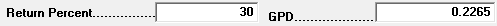GPD and Degree Day Routing Method

# GPD and Degree Day Routing Method

This routing method uses a combination of both the Gallons Per Day and the Gallons per Degree Day calculations to determine the next delivery date (assuming you have at least four deliveries for this tank). For instance, this might be used if a customer has both a water heater (where usage is consistent and Gallons Per Day is the best way to estimate usage) and a heating appliance (where Degree Days is more appropriate).

If you're unsure about either of these routing methods individually please see:

Example: The system will automatically calculate the K-factor (Gallons per Degree Day) the same as with the Degree Day method described in the overview article linked above, assuming you have at least four deliveries for this tank. You will then enter the Gallons Per Day and the Estimated Return Percent, like this:Note: If there is insufficient historical data (less than four deliveries) to automatically calculate the K-factor, use a different routing method.